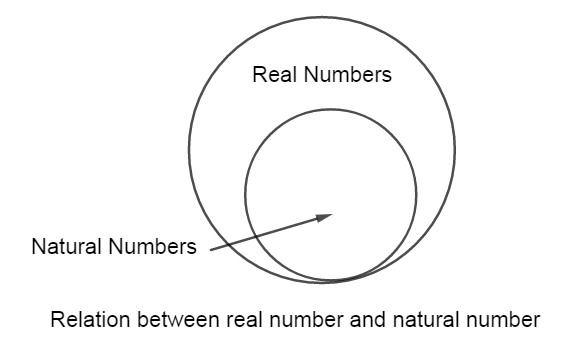QuestionAnswers

# What is the difference between Natural number and Real number?Hint: Firstly we have to know the definition of real number and natural numbers. Then we have to include the sets for real numbers and natural numbers. And conclude the difference with examples and a diagram of real and natural numbers.

Complete step by step solution:
We know that real numbers are simply the combination of rational and irrational numbers, in the number system. In general, all the arithmetic operations can be performed on these numbers and they can be represented in the number line, also. At the same time, the imaginary numbers are the un-real numbers, which cannot be expressed in the number line and is commonly used to represent a complex number. For example we can say integers (-2, 0, 1), fractions $\left( \dfrac{1}{2},2.5 \right)$ and irrational numbers such as $\sqrt{3},\sqrt{2},\pi$ etc.
On the other hand Natural numbers are a part of the number system which includes all the positive integers from 1 till infinity and are also used for counting purposes. It does not include zero (0). It can be written as
$\mathbb{N}=\left\{ 1,2,3,4........ \right\}$.
Which means All numbers such as 1, 2, 3, 4, 5, 6,……….
We can represent real number and natural number by this diagram,Where we can see natural numbers is part of the real number.
The set of real numbers consist of different categories, such as natural and whole numbers, integers, rational and irrational numbers. There are four main properties which include commutative property, associative property, distributive property and identity property.
Where for natural numbers there are four main properties which include Closure property, Commutative property, Associative property, Distributive property.

Note: To understand real numbers and natural numbers properly we have to understand the set theory of each number. This difference is incomplete without a diagram. We have to include examples for better understanding. Do not get confused with whole numbers and natural numbers, the difference between them is the element 0. Whole numbers contain 0 whereas natural numbers do not.

View Notes
Difference Between Log and LnCBSE Class 10 Maths Chapter 1 - Real Numbers FormulaDifference Between Dealer and DistributorDifference Between Ecosystem and BiomeDifference Between Environment and EcosystemDifference Between Chromatin and ChromosomesDifference between Cytoplasm and ProtoplasmDifference Between Respiration and CombustionWhat is the Difference Between Trade and Commerce?Important Questions for CBSE Class 10 Maths Chapter 1 - Real NumbersImportant Questions for CBSE Class 9 Maths Chapter 1 - Number SystemsImportant Questions for CBSE Class 9 Science Chapter 14 - Natural ResourcesCBSE Class 8 Science Some Natural Phenomena WorksheetsImportant Questions for CBSE Class 9 Maths Chapter 9 - Areas of Parallelograms and TrianglesImportant Questions for CBSE Class 7 Maths Chapter 9 - Rational NumbersNCERT Books for Class 9 Maths Chapter 9Important Questions for CBSE Class 9 MathsImportant Questions for CBSE Class 9 Maths Chapter 6 - Lines and AnglesImportant Questions for CBSE Class 9 Maths Chapter 13 - Surface Areas and VolumesCBSE Class 12 Maths Question Paper 2020CBSE Class 10 Maths Question Paper 2020Maths Question Paper for CBSE Class 10 - 2011Maths Question Paper for CBSE Class 10 - 2008CBSE Class 10 Maths Question Paper 2017Maths Question Paper for CBSE Class 10 - 2012Maths Question Paper for CBSE Class 10 - 2009Maths Question Paper for CBSE Class 10 - 2010Maths Question Paper for CBSE Class 10 - 2007Maths Question Paper for CBSE Class 12 - 2013RD Sharma Solutions for Class 9 Maths Chapter 2 - Exponents of Real NumbersNCERT Solutions for Class 10 Maths Chapter 1 Real NumbersRS Aggarwal Class 10 Solutions - Real NumbersRD Sharma Solutions for Class 10 Maths Chapter 1 - Real NumbersNCERT Solutions for Class 9 Science Chapter 14NCERT Solutions for Class 9 Maths Chapter 1 Number SystemRD Sharma Class 9 Solutions Chapter 2 - Exponents of Real Numbers (Ex 2.1) Exercise 2.1NCERT Solutions for Class 10 Maths Chapter 1 Real Numbers (Ex 1.1) Exercise 1.1NCERT Solutions for Class 10 Maths Chapter 1 Real Numbers (Ex 1.3) Exercise 1.3NCERT Exemplar for Class 10 Maths Chapter 1 - Real Numbers (Book Solutions)